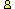CTK Exchange

 Subject: "infinity & probability" Previous Topic | Next Topic
 ConferencesThe CTK ExchangeGuest bookTopic #2300Printer-friendly copyEmail this topic to a friend Reading Topic #2300
eric derobert
Member since Nov-13-06
Nov-13-06, 07:03 AM (EST)"infinity & probability"

 This is just a remark about https://www.cut-the-knot.org/Probability/infinity.shtmlI agree that the individual probability for each ball to be in the urn is 0 (from the random process).But a probability for an event equal to 0 doesn't mean that this event never happen.For example, if you randomized a value between 0 and 1 according to an uniform distribution, the probability for each value to be chosen is 0, and nevertheless one value will have been chosen at the end of the story.In the "Infinity & Probability" story, the probability associated to each ball is then 0 ; but is the urn empty ?Best regards,Eric

alexbCharter Member
1917 posts
Nov-13-06, 07:35 AM (EST)1. "RE: infinity & probability"
In response to message #0

 >This is just a remark about >https://www.cut-the-knot.org/Probability/infinity.shtml Your remark caused me to check the page. There were several typos and worse. I am grateful for pushing me in the right direction.>But a probability for an event equal to 0 doesn't mean that >this event never happen. Agreed.>For example, if you randomized a value between 0 and 1 >according to an uniform distribution, the probability for >each value to be chosen is 0, and nevertheless one value >will have been chosen at the end of the story. >>In the "Infinity & Probability" story, the probability >associated to each ball is then 0 ; but is the urn empty ? It might not be. The page does not claim that it will but only that the probability of an empty urn is 1. I added a note to this effect.

 Conferences | Forums | Topics | Previous Topic | Next Topic
 Select another forum or conference Lobby The CTK Exchange (Conference)   |--Early math (Public)   |--Middle school (Public)   |--High school (Public)   |--College math (Public)   |--This and that (Public)   |--Guest book (Public) Educational Press (Conference)   |--No Child Left Behind (Public)   |--Math Wars (Public)   |--Mathematics and general education (Public)You may be curious to visit the old Guest book.